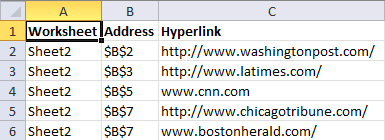Author: Oscar Cronquist Article last updated on September 20, 2017

The macro in this blog post lists all hyperlinks in a worksheet. It also lists all words beginning with "http" or "www". It grabs the hyperlink even if you have a hyperlink function in a cell.

Example, this sheet has two regular hyperlinks (B2:B3) and one hyperlink function (B5) and two web addresses (B7).This is what the macro returns, the worksheet name in column A. Cell address in column B and the hyperlink in column C.### VBA Macro

```Sub ListHyperlinks()
Set Asheet = ActiveSheet
Nsheet.Range("A1:C1").Font.Bold = True

i = 0
For Each cell In Asheet.UsedRange
On Error Resume Next
If Err = 0 Then
Nsheet.Range("A2").Offset(i, 0) = Asheet.Name
i = i + 1
Else
If Left(cell.Formula, 11) = "=HYPERLINK(" Then
strArray = Split(cell.Formula, Chr(34))
Nsheet.Range("A2").Offset(i, 0) = Asheet.Name
Nsheet.Range("C2").Offset(i, 0) = strArray(1)
i = i + 1
Else
strArray = Split(cell)
For Each vl In strArray
If Left(vl, 4) = "http" Or Left(vl, 3) = "www" Then
Nsheet.Range("A2").Offset(i, 0) = Asheet.Name
Nsheet.Range("C2").Offset(i, 0) = vl
i = i + 1
End If
Next vl
End If
End If
On Error GoTo 0
Next cell

Nsheet.Columns("A:C").AutoFit

End Sub
```

### Where to put the code?

1. Press Alt +F11
2. Insert a module
3. Paste vba code to code window
4. Exit VB Editor Alt + Q

### How to start macro?

1. Press Alt+F8
3. Click "Run" button

### What happens when I run the macro?

1. New sheet is inserted
2. Sheet is populated with hyperlink data

### How do I find all hyperlinks in a workbook?

This macro allows you to find links in all worksheets in active workbook.

```Sub ListHyperlinksInWB()

Nsheet.Range("A1:C1").Font.Bold = True

i = 0
For Each sh In ActiveWorkbook.Worksheets
If sh.Name <> Nsheet.Name Then

For Each cell In sh.UsedRange
On Error Resume Next
If Err = 0 Then
Nsheet.Range("A2").Offset(i, 0) = sh.Name
i = i + 1
Else
If Left(cell.Formula, 11) = "=HYPERLINK(" Then
strArray = Split(cell.Formula, Chr(34))
Nsheet.Range("A2").Offset(i, 0) = sh.Name
Nsheet.Range("C2").Offset(i, 0) = strArray(1)
i = i + 1
Else
strArray = Split(cell)
For Each vl In strArray
If Left(vl, 4) = "http" Or Left(vl, 3) = "www" Then
Nsheet.Range("A2").Offset(i, 0) = sh.Name
Nsheet.Range("C2").Offset(i, 0) = vl
i = i + 1
End If
Next vl
End If
End If
On Error GoTo 0
Next cell
End If
Next sh

Nsheet.Columns("A:C").AutoFit

End Sub

```